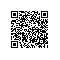# thinkphp模板中标签<volist>，<if>和 U函数的使用

<volist name="list" id="vo" empty="暂时没有数据" key="k">
<tr>
<td>{$k}</td> <td>{$vo.domain}</td>
<td>{$vo.contact}</td> <td>{$vo.ip}</td>
<td>
<if condition="$vo.status eq 1"> 有效 <else/> 无效 </if> </td> <td>{$vo.user_id}</td>
<td>{$vo.create_time}</td> <td><input type="checkbox" name="ids" class="j-icheck" value="1"></td> <td> <a href="{:U('Mess/form',array('id'=>$id))}" class="btn btn-green btn-sm" target="navTab" rel="form" reloadtips="本页已有打开的内容，确定将刷新本页内容，是否继续？">编辑</a>
</td>
</tr>
</volist>

<if condition="$vo.status eq 1"> 这里，$vo.status eq 1   不要加  { }

本文转自phpervip 51CTO博客，原文链接：http://blog.51cto.com/phpervip/1759911，如需转载请自行联系原作者使用钉钉扫一扫加入圈子
+ 订阅